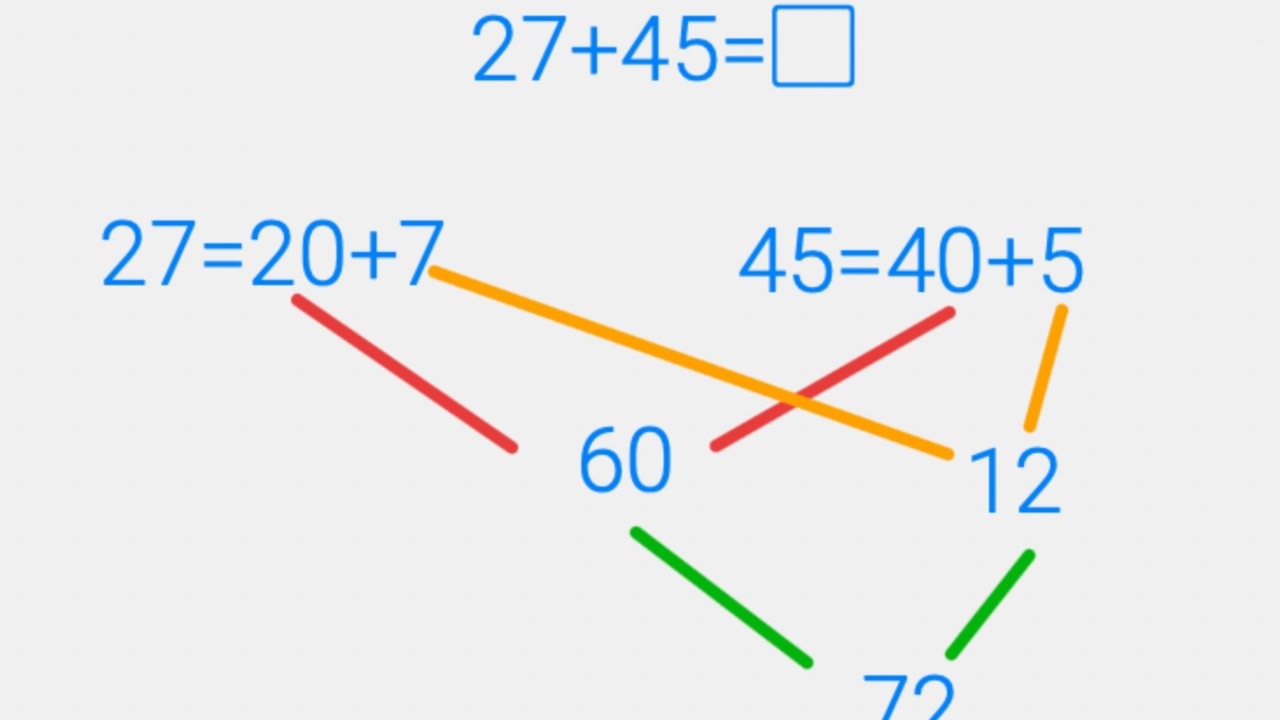# Addition Strategies - Partial Sums

Sep 22, 2022In second grade students are learning to add within 1000 using strategies based on place value.  (CCSS.MATH.CONTENT.2.NBT.B.7).  So what does that mean to use a strategy based on place value?  One strategy we can use is the partial sums addition strategy.

## Why use partial sums?

Once students understand how to use this strategy, it often becomes one that they use mentally to solve addition problems.  It eliminates the need to use small numbers at the top of the problem to indicate “carrying over” extra tens (or hundreds, etc.) and thus the logic of it is easier for students to follow than the standard algorithm for addition.

Let’s take a look at an example for 27+45.  Follow the steps below for first decomposing the number and then adding the tens and then the ones.

Here is another way to visualize this same strategy.

## Working With Larger Numbers

We can use the same strategy for adding larger numbers.  The third grade standards call for students to “Fluently add and subtract within 1000 using strategies based on place value.” (CCSS.MATH.CONTENT.3.NBT.A.2). When we use the partial sums addition strategy, we are preserving the place value of the numbers and thinking in groups of hundreds, tens, and ones. Preserving the place value of the numbers makes it easier to follow the logic of the problem and thus makes it a strategy that students can use efficiently. We can use it for even larger numbers too. Take a look at this example using numbers in the thousands.

So now think about your own mind.  If you needed to add 427 + 172=____ would you rather grab a piece of paper and a pencil and use the standard algorithm or could you easily see adding the hundreds, then the tens, and then the ones?  The more you practice the partial sums addition strategy, the easier it gets. The standard algorithm is likely the strategy you were taught in school and you practiced it over and over so it seems the easiest. However, I can attest that partial sums is now my "go-to" strategy after practicing it with students. I think you will come to appreciate it too after some practice. It has the added bonus of being an easy strategy to use mentally – no paper or pencil needed.